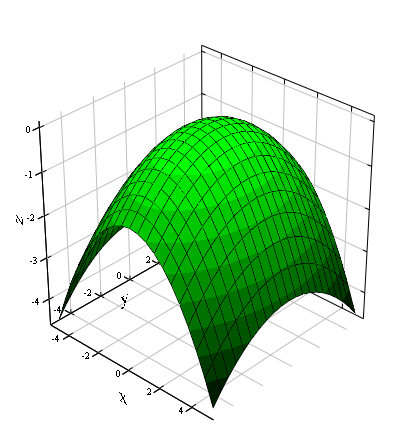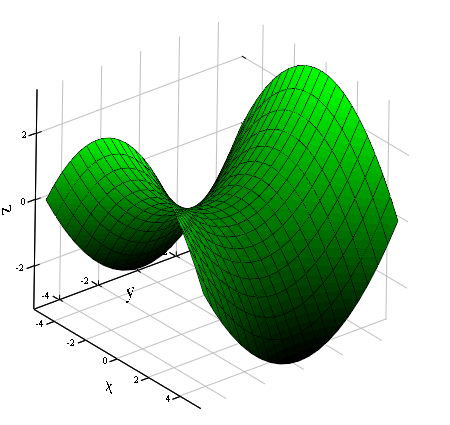# How to parameterize an elliptical paraboloid

## Paraboloids

A Paraboloid is a 2nd order surface.A distinction is made between an elliptical and a hyperbolic paraboloid. The elliptical paraboloid resembles - depending on the strength of the curvature - a bowl or a cup. The hyperbolic paraboloid is a saddle surface.
The formula for a paraboloid with the axis in the z-direction is:
• elliptical paraboloid: a2x2 + b2y2 −z = 0
• hyperbolic paraboloid: a2x2 −b2y2 −z = 0
If a paraboloid is cut from a plane perpendicular to its axis, the sectional image is an ellipse or hyperbola. A plane section parallel to the axis results in a parabola.
An elliptical paraboloid with a = b is also known as a paraboloid of revolution.

### Formulas

The formulas apply to a paraboloid of revolution that is cut off by a plane perpendicular to the z-axis (xy plane) at height h. The circle of intersection has the radius r.
Volume: V = 2π ⋅r2⋅h
Surface (without covering circle area): AO = 6h2πr ⋅⎣⎢⎢⎡ (r2 + 4h2) 23 −r3⎦⎥⎥⎤
Height of the center of gravity: hS = 32 ⋅h

### Hyperbolic paraboloidHyperbolic paraboloid
The hyperbolic paraboloid From a mathematical point of view, (saddle surface) is a surface of the second order. This means that every section with a plane results in a conic section. In this case they are:
• for vertical planes: parabolas
• for horizontal or any inclined planes: hyperbolas
Such a surface is also called an anticlastic (= oppositely curved) surface.
The equation of the hyperbolic paraboloid is: a2x2 −b2y2 −2z = 0
The surface can be created by moving a hanging parabola (open at the bottom) along a standing parabola that is open at the top. It is interesting that it can, however, also be represented by two sets of straight lines. This means that the hyperbolic paraboloid can be constructed from rectilinear elements (ropes, steel girders).